Moving Average Trading Strategies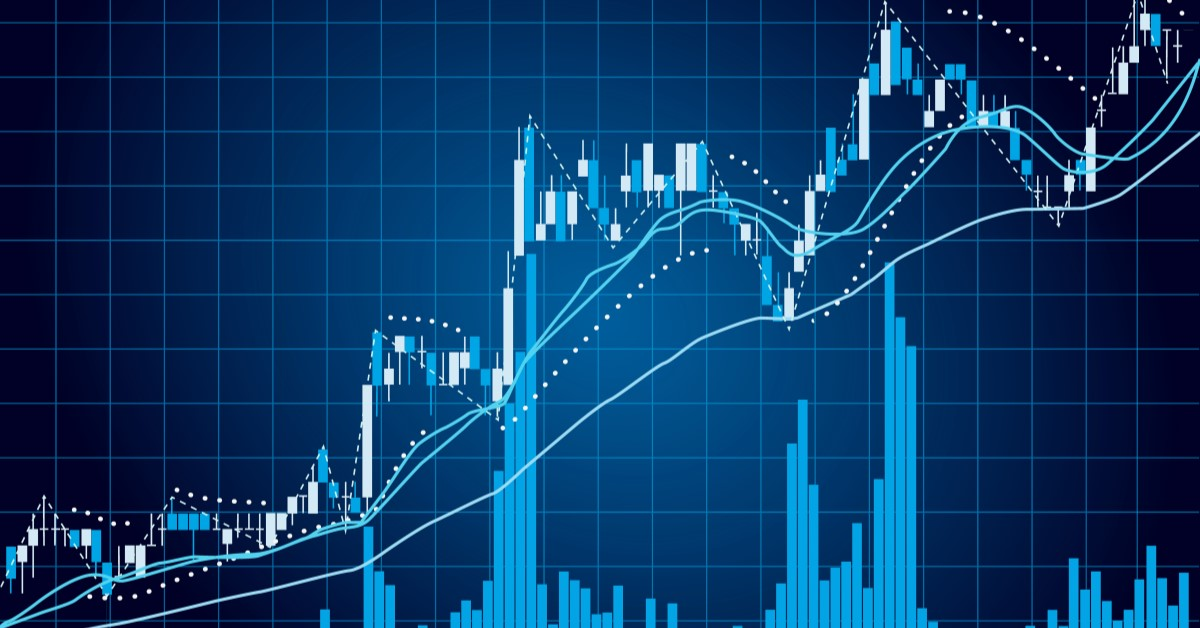What are Moving Averages?

Moving average is one of the most widely used technical indicators for validating the movement of markets. Few other indicators have proved to be as unbiased, definitive and practical as the moving average. Moving averages help traders identify trends and empower them to increase the number of profitable trades by making those trends work in their favour.

Moving averages (occasionally referred in this blog post as MA) are the averages of a series of numeric values. They have a predefined length for the number of values to average and this set of values moves forward as more data is added with time. Given a series of numbers and a fixed subset size, the first element of the MA series is obtained by taking the average of the initially fixed subset of the number series. The subset is then modified by shifting it forwards by one value, i.e. by excluding the first element of the previous subset and adding the element immediately after the previous subset to the new subset keeping the length fixed. Consider the example mentioned below to understand the calculation of simple moving averages. Let the average be calculated for five data points (underline denotes the subset used for calculating the average).

Number series:  7  12  2  14  15  16  11  20  7

1st value of the MA series:  (7 + 12 + 2 + 14 + 15) / 5 = 10             7  12  2  14  15  16  11  20  7

2nd value of the MA series:  (12 + 2 + 14 + 15 + 16) / 5 = 11.8       7  12  2  14  15  16  11  20  7

3rd value of the MA series:  (2 + 14 + 15 + 16 + 11) / 5 = 11.6         7  12  2  14  15  16  11  20  7

It can be seen that the subset for calculating averages moves forward by one data entry, consequently the name moving average (also called running average or rolling average). A moving average series can be calculated for any time series. In financial markets, it is most often applied to stock and derivative prices, percentage returns, yields and trading volumes. The price of securities tend to fluctuate rapidly, as a result the graphs contain several peaks and troughs making it difficult to understand the overall movement. Moving averages help smoothen out the fluctuations, enabling analysts and traders to predict the trend or movement in the price of securities. Larger subsets for calculating moving averages will generate smoother curves and contain lesser fluctuations. This happens as each data point in the subset has lesser weightage when the lookback period is increased, which in turn reduces the variations inherent in the underlying price/volume chart. These moving averages are slower to respond to a change in trend and are called slow moving averages. The moving averages with shorter durations are known as fast moving averages and are faster to respond to a change in trend. Slow moving averages are also called larger moving averages as they have a larger subset for computing the average. Similarly fast moving averages are also called smaller moving averages. Moving averages are known to be lagging indicators, they lag behind movements in the price/volume charts. A faster MA has less lag when compared to the slower MA.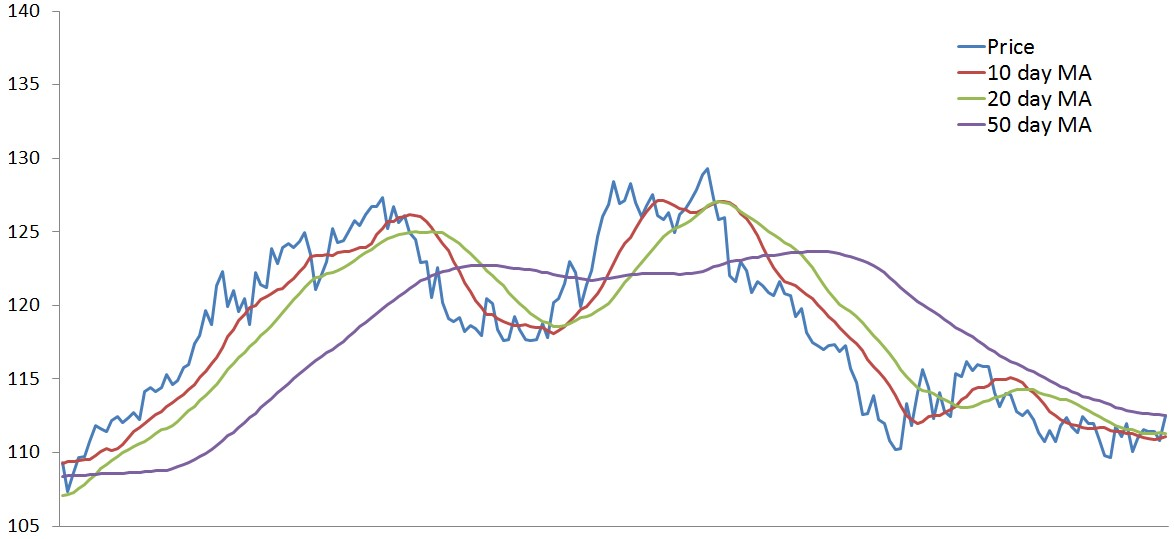Consider the chart shown above, it contains the closing price of a futures contract (blue line), the 10 day moving average (red line), the 20 day moving average (green line) and the 50 day moving average (purple line). It can be observed that the 50 day MA is the smoothest and the 10 day MA has the maximum number of peaks and troughs or fluctuations. As the lookback period increases, the moving average line moves away from the price curve. The red line (10 day MA) is closest to the blue line (price curve) and the purple line (50 day MA) is farthest away. If one were to shift the MA lines in order to overlap them with the price curve, the shift would have to be made in the direction of negative x-axis, this confirms the lagging property of the MA lines. The 50 day MA would require the maximum shift, meaning that slower moving averages have greater lag than the faster moving averages. The slower moving average is slower in responding to changes in the price curve.

There are different types of moving averages that can be used to develop a vast variety of moving average strategies, let us now look at a few of these in more detail.

Types of Moving Averages

There are many different types of moving averages depending on the computation of the averages. The five most commonly used types of moving averages are the simple (or arithmetic), the exponential, the weighted, the triangular and the variable moving average. The only noteworthy difference between the various moving averages is the weight assigned to data points in the moving average period. Simple moving averages apply equal weight to all data points. Exponential and weighted averages apply more weight to recent data points. Triangular averages apply more weight to data in the middle of the moving average period. The variable moving average changes the weight based on the volatility of prices.

1. Simple Moving Average (SMA)

A simple (or arithmetic) moving average is an arithmetic moving average calculated by adding the elements in a time series and dividing this total by the number of time periods. As the name suggests, the simple moving average is the simplest type of moving average. It is arguably the most popular technical analysis tool used by traders. All elements in the SMA have the same weightage. If the moving average period is 5, then each element in the SMA will have a 20% (1/5) weightage in the SMA. The SMA is usually used to identify trend direction, but it can also be used to generate potential trading signals. The formula for calculating the SMA is straightforward:
SMA = (Sum of data points in the moving average period)/(Total number of periods)

2. Exponential Moving Average (EMA or EWMA)

The simple moving averages are sometimes too simple and do not work well when there are spikes in the price of the security. Exponential moving averages give more weight to the most recent periods. This makes them more reliable than the SMA and a better representation of the recent performance of the security and hence can be used to create a better moving average strategy. The EMA is calculated as shown below:
Weighting multiplier = 2 / (moving average period +1)

EMA = (Closing price - EMA of previous day/bar) x multiplier) + EMA of previous day/bar

Rewritten as:

EMA = (Closing price) x multiplier + (EMA of previous day/bar) x (1 - multiplier)

The weightage to the most recent data is greater for a shorter period EMA than a longer period EMA. For example, a 10 period EMA applies a weightage of 18.18% (2/11), whereas that for a 20 period EMA is 9.52% (2/21). The name exponential moving average is because each term in the moving average period has an exponentially greater weightage than its preceding term. The exponential moving average is faster to react than the simple moving average, this can be seen in the chart below (blue line represents the daily closing price, red line represents the 30 day SMA and the green line represents the 30 day EMA).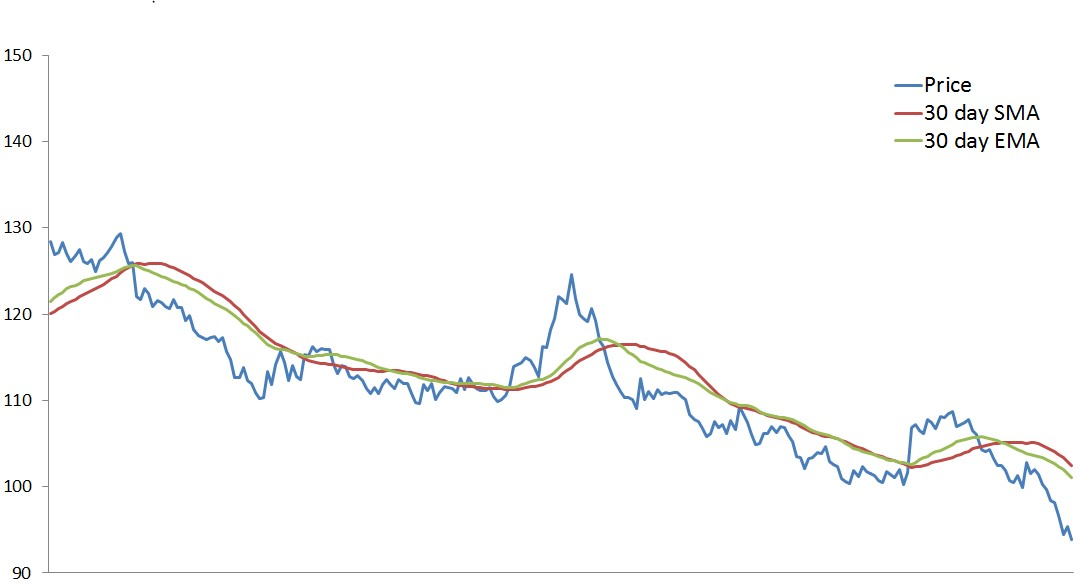The following extract from John J. Murphy’s work, “Technical Analysis of the Financial Markets” published by the New York Institute of Finance in 1999, contains one of the best explanations about the advantage of the exponentially weighted moving average over the simple moving average.

“The exponentially smoothed moving average addresses both of the problems associated with the simple moving average. First, the exponentially smoothed average assigns a greater weight to the more recent data. Therefore, it is a weighted moving average. But while it assigns lesser importance to past price data, it does include in its calculation all the data in the life of the instrument. In addition, the user is able to adjust the weighting to give greater or lesser weight to the most recent day's price, which is added to a percentage of the previous day's value. The sum of both percentage values adds up to 100.”

3. Weighted Moving Average (WMA or LWMA)

The weighted moving average refers to the moving averages where each data point in the moving average period is given a particular weightage while computing the average. The exponential moving average is a type of weighted moving average where the elements in the moving average period are assigned an exponentially increasing weightage. A linearly weighted moving average (LWMA), also generally referred to as weighted moving average (WMA), is computed by assigning a linearly increasing weightage to the elements in the moving average period.

If the moving average period contains ten data entries, then the most recent element (the tenth element) will be multiplied by ten, the ninth element will be multiplied by nine and so on till the first element which will have a multiplier of one. The sum of all these linearly weighted elements will then be added and divided by the sum of the multipliers, in case of 10 elements the sum will be divided by 55 (n(n+1)/2). The chart shown below plots the SMA (red line), EMA (green line) and LWMA (purple line) for a 30 day period.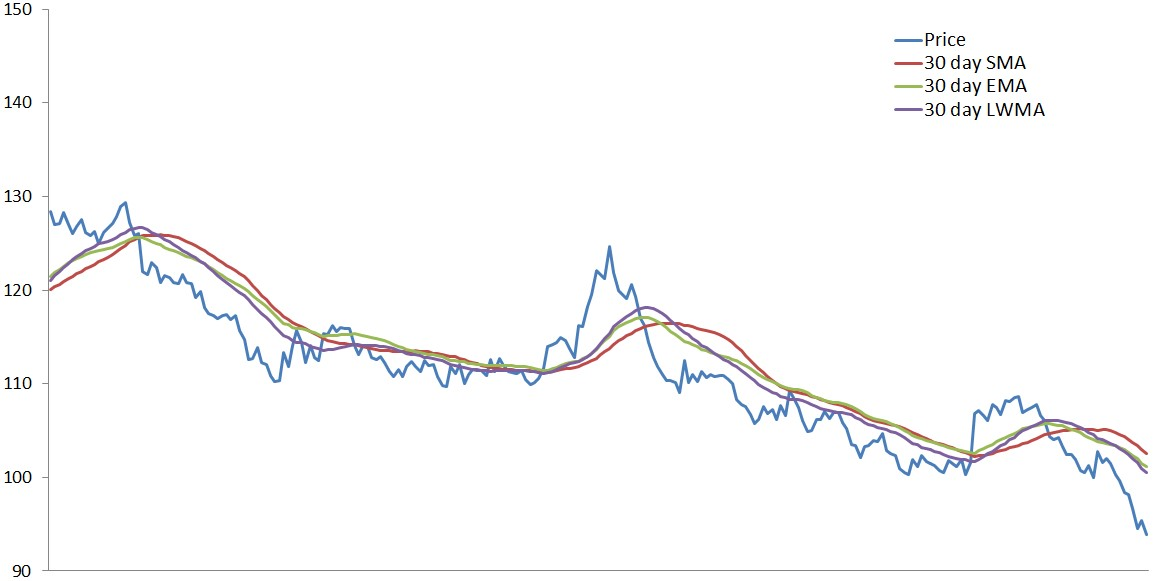As it can be seen in the chart above that like the exponential moving average, the weighted moving average is faster to respond to changes in the price curve than the simple moving average, but it is slightly slower to react to fluctuations than the EMA this is because the LWMA lays slightly greater stress on the recent past data than the EMA, which applies a weightage to all previous data in an exponentially decreasing manner. Mentioned below are the weightage given to elements when calculating the EMA and WMA for a 4 day period:

EWMA                                                         LWMA

Most recent element:                     2/(4+1) = 40%                                                4/10 = 40%

2nd most recent element:           40% x 60% = 24%                                         3/10 = 30%

3rd most recent element:            24% x 60% = 14.4%                                       2/10 = 20%

4th most recent element:            14.4% x 60% = 8.6%                                      1/10 = 10%

5th most recent element:            8.6% x 60% = 5.2%                                       0/10 = 0%

6th most recent element:            5.2% x 60% = 3.1%                                         0/10 = 0%

7th most recent element:            3.1% x 60% = 1.9%                                          0/10 = 0%

And so on...

4. Triangular Moving Average (TMA)

The triangular moving average is a double smoothed curve, which also means that the data is averaged twice (by averaging the simple moving average). TMA is a type of weighted moving average where the weightage is applied in a triangular pattern. Follow the steps mentioned below to compute the TMA:

First, calculate the simple moving average (SMA):

SMA = (D1 + D2 + D3 + . . . . . . + Dn) / n
Next calculate the average of the SMAs:
TMA = (SMA1 + SMA2 + SMA3 + . . . . . . + SMAn) / n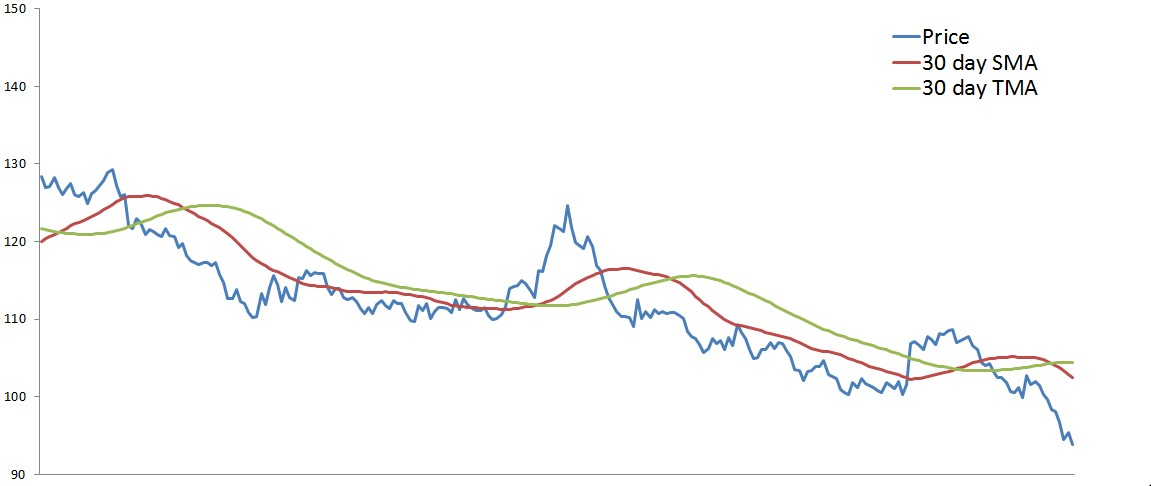Consider the chart shown above, which comprises of the daily closing price curve (blue line), the 30 day SMA (red line) and the 30 day TMA (green line). It can be observed that the TMA is much smoother than the SMA. The TMA moves in longer and steadier waves than the SMA. The lag in TMA is greater than other moving averages, like the SMA and the EMA, because of the double averaging. It can be observed that the TMA takes longer to react to price fluctuations. The trading signals generated by the TMA during a trending period will be farther away from the peak and trough of the period when compared to the ones generated by the SMA, hence lesser profits will be made by using the TMA. However, during a consolidation period, the TMA will not produce as many unavailing trading signals as those generated by the SMA, which would avoid the trader from taking unnecessary positions reducing the transaction costs.

5. Variable Moving Average (VMA)

The variable moving average is an exponentially weighted moving average developed by Tushar Chande in 1991. Chande suggested that the performance of an exponential moving average could be improved by using a Volatility Index (VI) to adjust the smoothing period when market conditions change. Volatility is the measure of how quickly or slowly prices change over time.  The volatility index shows the market’s volatility expectations for the next 30 days. The purpose of developing the VMA was to slow down the average when prices are in the consolidation period to avoid unavailing trading signals and to speed up the average when the market is trending so as to make the most out of the trending prices. Given below is the method for calculating the variable moving average: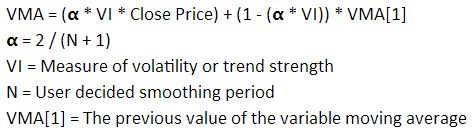Moving Average Trading Strategies

1. Triple Moving Average Crossover Strategy

The triple moving average strategy involves plotting three different moving averages to generate buy and sell signals. This moving average strategy is better equipped at dealing with false trading signals than the dual moving average crossover system. By using three moving averages of different lookback periods, the trader can confirm whether the market has actually witnessed a change in trend or whether it is only resting momentarily before continuing in its previous state. The buy signal is generated early in the development of a trend and a sell signal is generated early when a trend ends. The third moving average is used in combination with the other two moving averages to confirm or deny the signals they generate. This reduces the probability that the trader will act on false signals.

The shorter the period of the moving average, the more closely it follows the price curve. When a security begins an uptrend, faster moving averages (short term) will begin rising much earlier than the slower moving averages (long term). Assume that a security has risen by the same amount each day for the last 60 trading days and then begins to decline by the same amount for the next 60 days. The 10 day moving average will start declining on the sixth trading day, the 20 day and 30 day moving averages will start their decline on the eleventh and the sixteenth day respectively. The probability of a trend to persist is inversely related to the time that the trend has already persisted. Because of this reason, waiting to enter a trade for too long results in missing out on most of the gain, whereas entering a trade too early can mean entering on a false signal and having to exit the position at a loss. To address this issue, traders use the triple moving average crossover strategy aiming to ride the trend for just the right time and avoiding false signals while doing so.

To illustrate this moving average strategy we will use the 10 day, 20 day and 30 day simple moving averages as plotted in the chart below. The duration and type of moving averages to be used depends on the time frames that the trader is looking to trade in. For shorter time frames (one hour bars or faster), exponential moving average is preferred due its tendency to follow the price curve closely (e.g. 4, 9, 18 EMA or 10, 25, 50 EMA). For longer time frames (daily or weekly bars), traders prefer using simple moving averages (e.g. 5, 10, 20 SMA or 4, 10, 50 SMA). The moving average periods vary depending on the trader’s strategy and the security being traded.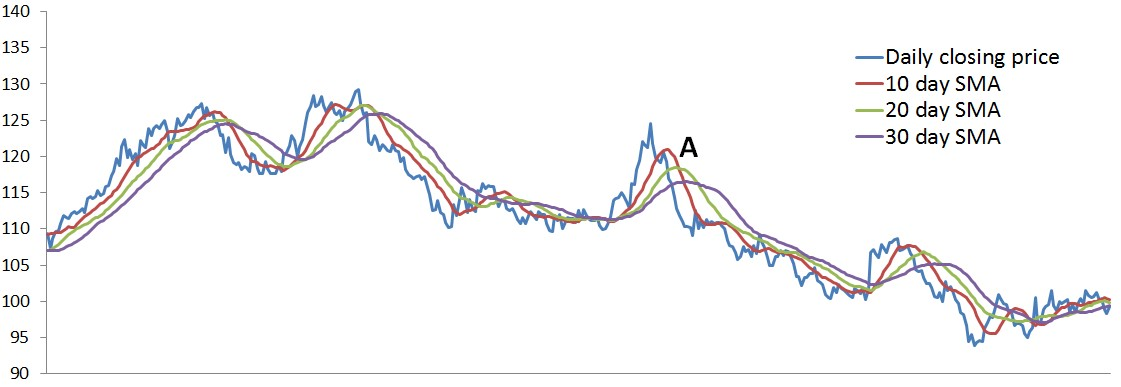Consider point ‘A’ on the chart above, the three moving averages change direction around this point. The red line represents the fast moving average (10 day SMA),  the green line represents the medium moving average (20 day SMA) and the purple line represents the slow moving average (30 day SMA). A signal to sell is triggered when the fast moving average crosses below both the medium and the slow moving averages. This shows a short term shift in the trend, i.e. the average price over the last 10 days has fallen below the average price of the last 20 and 30 days. The signal to sell is confirmed when the medium moving average crosses below the slow moving average, the shift in momentum is considered to be more significant when the medium (20 day) moving average crosses below the slow (30 day) moving average. The triple moving average crossover system generates a signal to sell when the slow moving average is above the medium moving average and the medium moving average is above the fast moving average. When the fast moving average goes above the medium moving average, the system exits its position. For this reason, unlike the dual moving average trading system, the triple moving average system is not always in the market. The system is out of the market when the relationship between the slow and medium moving average does not match that between the medium and fast moving averages.

More aggressive traders would not wait for the confirmation of the trend and instead enter into a position based on the fast moving average crossing over the slow and medium moving averages. One may also enter positions at different times, for example: the trader could take a certain number of long positions when the fast MA crosses above the medium MA, then take up the next set of long positions when the fast MA crosses above the slow MA and finally more long positions when the medium crosses over the slow MA. If at anytime a reversal of trend is observed he may exit his positions.

2. Moving Average Ribbon

An extended version of the moving average crossover system is the Moving Average Ribbon. This moving average strategy is created by placing a large number of moving averages onto the same chart (the chart shown below uses 8 simple moving averages). One must factor the time horizons and investment objectives while selecting the lengths and type of moving averages. When all the moving averages are moving in the same direction, the trend is said to be strong. Trading signals are generated in a similar manner to the triple moving average crossover system, the trader must decide the number of crossovers to trigger a buy or sell signal. Traders look to buy when the faster moving averages cross above the slower moving averages and look to sell when the faster moving averages cross below the slower moving averages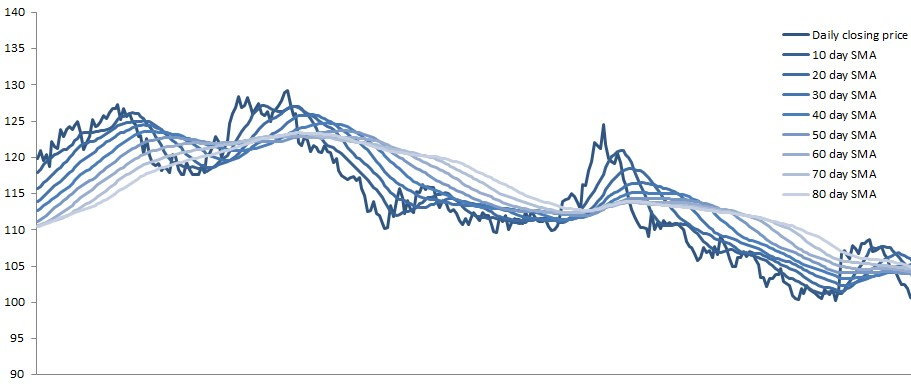3. Moving Average Convergence Divergence (MACD)

The MACD, short for moving average convergence divergence, is a trend following momentum indicator. It is a collection of three time series calculated as moving averages from historical price data, most often closing price. The MACD line is the difference between a fast (short term) exponential moving average and a slow (long term) exponential moving average of the closing price of a particular security. The signal line is the exponential moving average of the MACD line. In this moving average strategy, the trader looks for crossovers between the MACD and the signal line.

The MACD strategy is denoted by the three parameters which define the strategy, i.e. the time periods of the three moving averages - MACD(a,b,c), where the MACD series is the difference between EMAs with time periods ‘a’ and ‘b’. The signal line, which is the EMA of the MACD series has a time period of ‘c’. The most commonly used MACD strategy uses the 12 day and 26 day EMA for the MACD series and a 9 day EMA for the signal series, represented by MACD(12, 26, 9). The chart shown below is plotted based on these input parameters

MACD line = 12 day EMA of  closing price - 26 day EMA of closing price

Signal line = 9 day EMA of MACD line

Histogram = MACD line - Signal line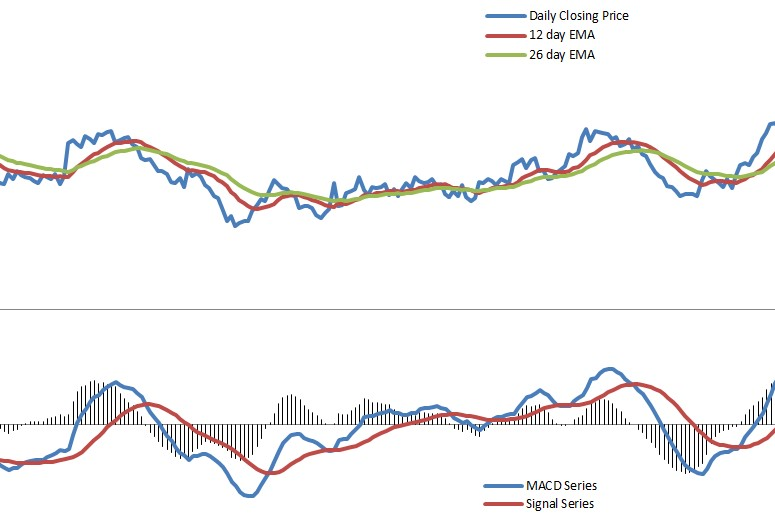The upper half of the chart contains the daily closing price (blue line), 12 day EMA (red line) and the 26 day EMA (green line). The lower half of the chart consists of the MACD Series (blue line), which is calculated by the subtracting the slow moving average (26 day EMA) from the fast moving average (12 day EMA), the Signal Series (red line) calculated by taking a 9 day EMA of the MACD Series and lastly the MACD histogram (black vertical lines), which is plotted by subtracting the Signal Series from the MACD Series.

There are many different interpretations of the MACD chart. The most commonly used signal trigger is when the MACD line crosses over the Signal line. When the MACD line crosses above the signal line, it is recommended to buy the underlying security and when the MACD line crosses below the signal line, a signal to sell is triggered. These events are taken as signs that the trend in the underlying security is about to escalate in the direction of the crossover. Another crossover that is taken into consideration by traders is called the zero crossover. This occurs when the slow and fast moving averages of the price curve crossover each other, or when the MACD series changes sign. A change from positive to negative is considered to be a bearish sign while a change from negative to positive is considered as a bullish sign. The zero crossover provides confirmation about a change in trend but it is less reliable in triggering signals than the signal crossover. Traders also monitor the divergence between the MACD line and the signal line, which can be observed through the histogram. When the histogram starts falling (moves towards the zero line), it indicates that the trend is weakening, this happens when the MACD and signal lines are converging. Whereas, when the signal line and MACD line are diverging, or the histogram is rising (moves away from the zero line), it is an indication that the trend is growing stronger.

Like other moving average strategies which are used for forecasting trends, the MACD can generate false signals. A bullish or bearish crossover followed by a sudden decline or rise in the underlying security respectively is called a false positive. A false negative is when there is no crossover yet the stocks suddenly accelerates either upwards or downwards.

Next Steps

Watch this informative session on 'Momentum Based Strategies' focused on the various aspects of Conventional/Low Frequency as well as High Frequency (HFT). In addition to this, you can check our blog for articles on different quantitative trading strategies.

Disclaimer: All investments and trading in the stock market involve risk. Any decisions to place trades in the financial markets, including trading in stock or options or other financial instruments is a personal decision that should only be made after thorough research, including a personal risk and financial assessment and the engagement of professional assistance to the extent you believe necessary. The trading strategies or related information mentioned in this article is for informational purposes only.